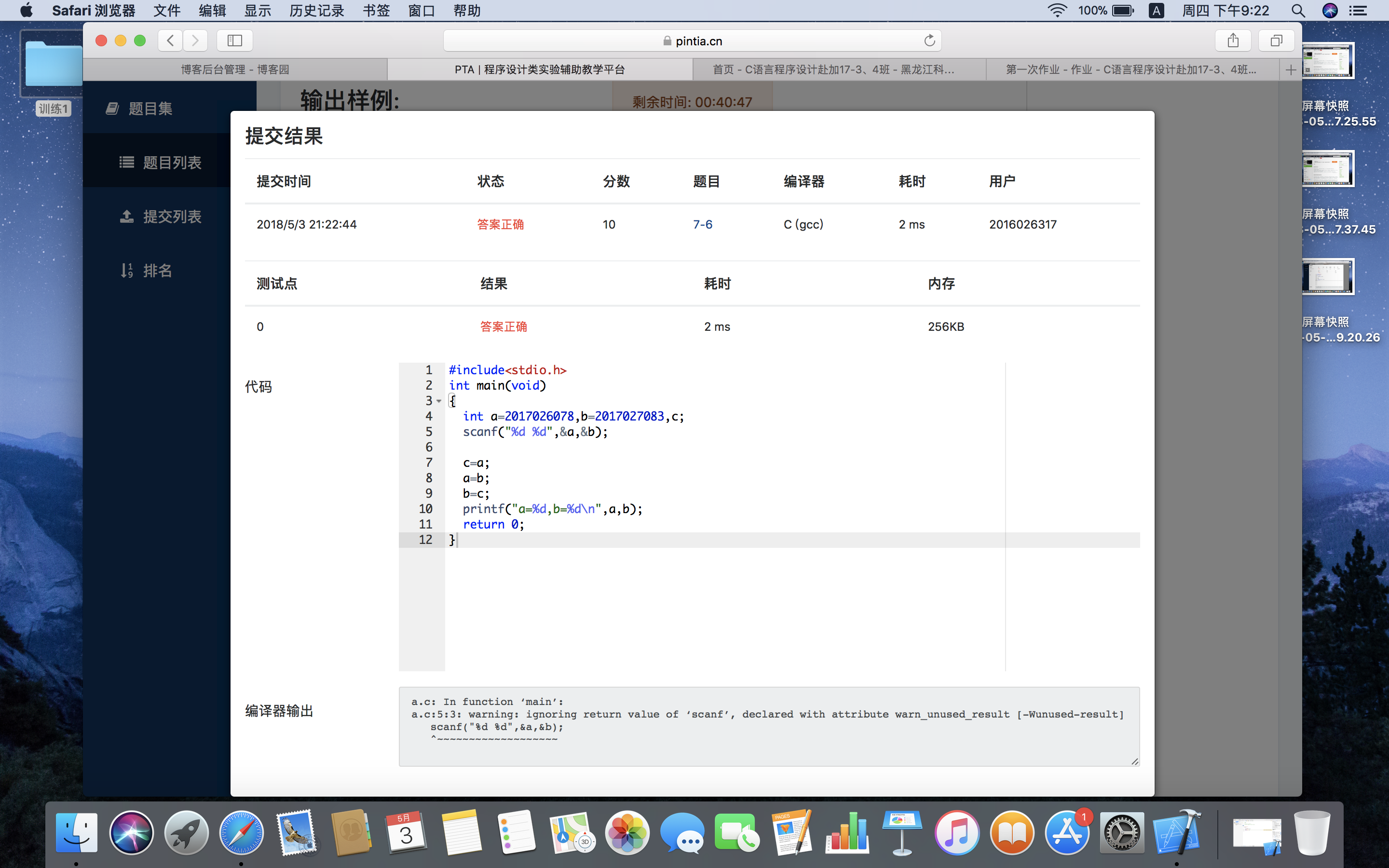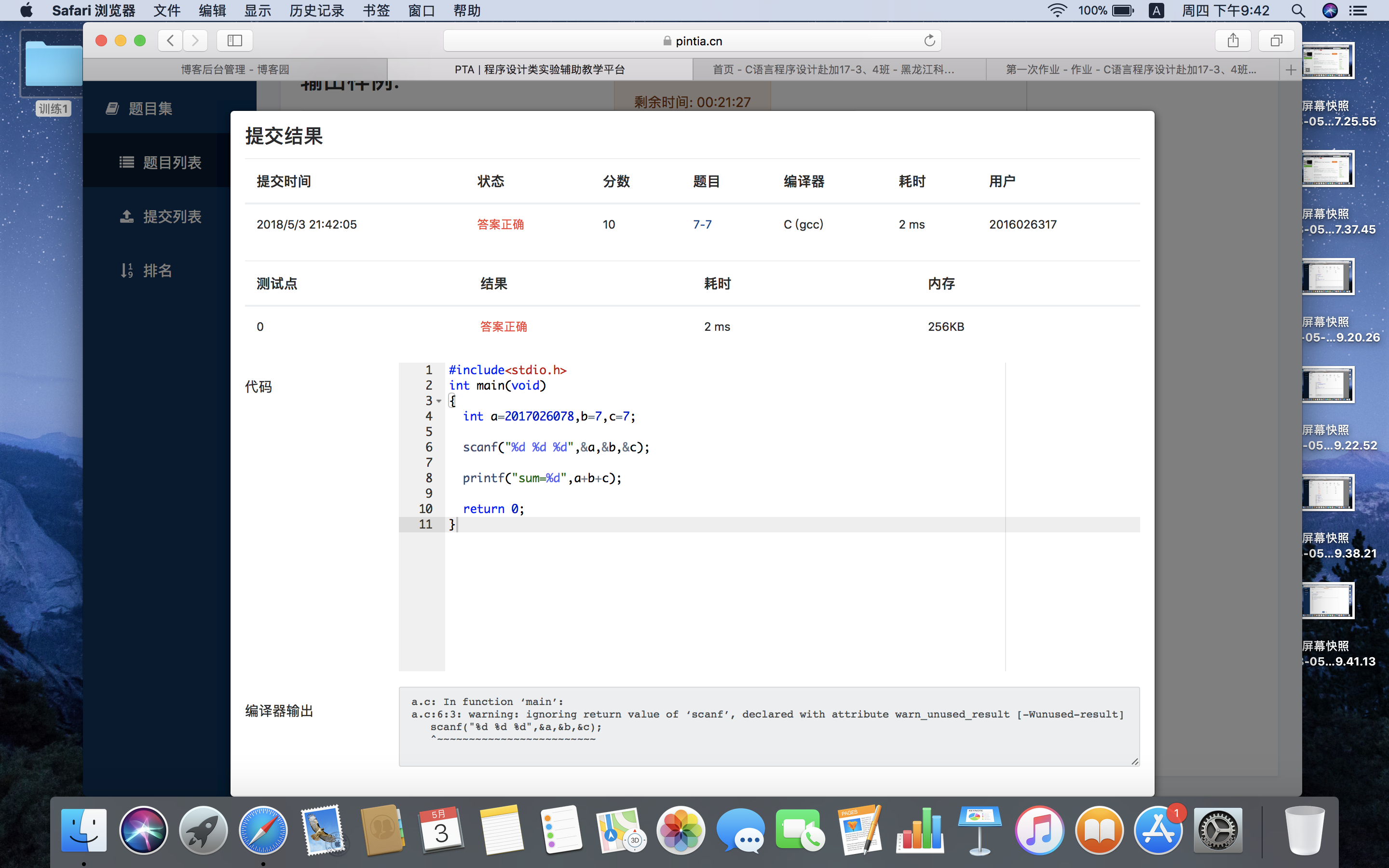# 第一次作业 17-3吕行

2:问题二：while，do while，for的转换

3:问题三：循环结构程序的设计

#include<stdio.h>
int main(void)
{
int a=2017026078,b=2017027083,c;
scanf("%d %d",&a,&b);

c=a;
a=b;
b=c;
printf("a=%d,b=%d\n",a,b);
return 0;
}2:7-7

#include<stdio.h>
int main(void)
{
int a=2017026078,b=7,c=7;

scanf("%d %d %d",&a,&b,&c);

printf("sum=%d",a+b+c);

return 0;
}3:7-3

#include<stdio.h>
int main (void)
{
int a,b;
scanf("%d",&a);
if(a<=5){
b=a+2;
printf("%d\n",b);
}
else{
b=a+2-7;
printf("%d\n",b);
}
return 0;
}

四：posted on 2018-05-03 21:53  -行止  阅读(190)  评论(0编辑  收藏  举报